Search by Topic

Resources tagged with Addition & subtraction similar to One Wasn't Square:

Filter by: Content type:
Age range:
Challenge level:

There are 215 results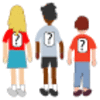One Wasn't Square

Age 7 to 11 Challenge Level:

Mrs Morgan, the class's teacher, pinned numbers onto the backs of three children. Use the information to find out what the three numbers were.Age 5 to 11 Challenge Level:

Try out this number trick. What happens with different starting numbers? What do you notice?The Clockmaker's Birthday Cake

Age 7 to 11 Challenge Level:

The clockmaker's wife cut up his birthday cake to look like a clock face. Can you work out who received each piece?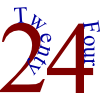The 24 Game

Age 7 to 11 Challenge Level:

There are over sixty different ways of making 24 by adding, subtracting, multiplying and dividing all four numbers 4, 6, 6 and 8 (using each number only once). How many can you find?Sam's Quick Sum

Age 7 to 11 Challenge Level:

What is the sum of all the three digit whole numbers?Oddly

Age 7 to 11 Challenge Level:

Find the sum of all three-digit numbers each of whose digits is odd.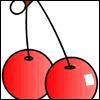Cherries Come in Twos

Age 7 to 11 Challenge Level:

Susie took cherries out of a bowl by following a certain pattern. How many cherries had there been in the bowl to start with if she was left with 14 single ones?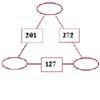Number Juggle

Age 7 to 11 Challenge Level:

Fill in the missing numbers so that adding each pair of corner numbers gives you the number between them (in the box).The Deca Tree

Age 7 to 11 Challenge Level:

Find out what a Deca Tree is and then work out how many leaves there will be after the woodcutter has cut off a trunk, a branch, a twig and a leaf.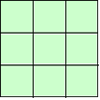1, 2, 3 Magic Square

Age 7 to 11 Challenge Level:

Arrange three 1s, three 2s and three 3s in this square so that every row, column and diagonal adds to the same total.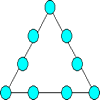Magic Triangle

Age 7 to 11 Challenge Level:

Place the digits 1 to 9 into the circles so that each side of the triangle adds to the same total.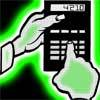Reverse Trick

Age 7 to 11 Challenge Level:

Tell your friends that you have a strange calculator that turns numbers backwards. What secret number do you have to enter to make 141 414 turn around?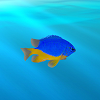Sea Level

Age 7 to 11 Challenge Level:

The picture shows a lighthouse and many underwater creatures. If you know the markings on the lighthouse are 1m apart, can you work out the distances between some of the different creatures?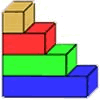Rod Measures

Age 7 to 11 Challenge Level:

Using 3 rods of integer lengths, none longer than 10 units and not using any rod more than once, you can measure all the lengths in whole units from 1 to 10 units. How many ways can you do this?Napier's Bones

Age 7 to 11 Challenge Level:

The Scot, John Napier, invented these strips about 400 years ago to help calculate multiplication and division. Can you work out how to use Napier's bones to find the answer to these multiplications?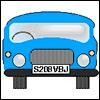Numbered Cars

Age 7 to 11 Challenge Level:

I was looking at the number plate of a car parked outside. Using my special code S208VBJ adds to 65. Can you crack my code and use it to find out what both of these number plates add up to?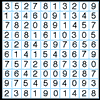A Numbered Route

Age 7 to 11 Challenge Level:

Can you draw a continuous line through 16 numbers on this grid so that the total of the numbers you pass through is as high as possible?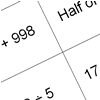How Do You Do It?

Age 7 to 11 Challenge Level:

This group activity will encourage you to share calculation strategies and to think about which strategy might be the most efficient.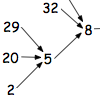Age 7 to 11 Challenge Level:

What happens when you add the digits of a number then multiply the result by 2 and you keep doing this? You could try for different numbers and different rules.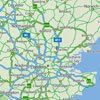Journeys

Age 7 to 11 Challenge Level:

Investigate the different distances of these car journeys and find out how long they take.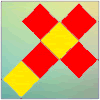Let's Face It

Age 7 to 11 Challenge Level:

In this problem you have to place four by four magic squares on the faces of a cube so that along each edge of the cube the numbers match.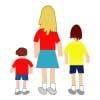Sisters and Brothers

Age 7 to 11 Challenge Level:

Cassandra, David and Lachlan are brothers and sisters. They range in age between 1 year and 14 years. Can you figure out their exact ages from the clues?I'm Eight

Age 5 to 11 Challenge Level:

Find a great variety of ways of asking questions which make 8.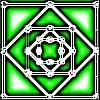Number Squares

Age 5 to 11 Challenge Level:

Start with four numbers at the corners of a square and put the total of two corners in the middle of that side. Keep going... Can you estimate what the size of the last four numbers will be?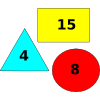Super Value Shapes

Age 7 to 11 Challenge Level:

If each of these three shapes has a value, can you find the totals of the combinations? Perhaps you can use the shapes to make the given totals?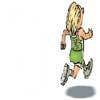Rocco's Race

Age 7 to 11 Short Challenge Level:

Rocco ran in a 200 m race for his class. Use the information to find out how many runners there were in the race and what Rocco's finishing position was.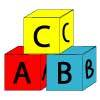Alphabet Blocks

Age 5 to 11 Challenge Level:

These alphabet bricks are painted in a special way. A is on one brick, B on two bricks, and so on. How many bricks will be painted by the time they have got to other letters of the alphabet?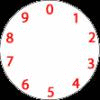Dividing a Cake

Age 7 to 11 Challenge Level:

Annie cut this numbered cake into 3 pieces with 3 cuts so that the numbers on each piece added to the same total. Where were the cuts and what fraction of the whole cake was each piece?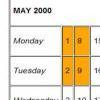Calendar Patterns

Age 7 to 11 Challenge Level:

In this section from a calendar, put a square box around the 1st, 2nd, 8th and 9th. Add all the pairs of numbers. What do you notice about the answers?Age 7 to 11 Challenge Level:

Can you put plus signs in so this is true? 1 2 3 4 5 6 7 8 9 = 99 How many ways can you do it?A-magical Number Maze

Age 7 to 11 Challenge Level:

This magic square has operations written in it, to make it into a maze. Start wherever you like, go through every cell and go out a total of 15!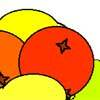Oranges and Lemons

Age 7 to 11 Challenge Level:

On the table there is a pile of oranges and lemons that weighs exactly one kilogram. Using the information, can you work out how many lemons there are?Calendar Calculations

Age 7 to 11 Challenge Level:

Try adding together the dates of all the days in one week. Now multiply the first date by 7 and add 21. Can you explain what happens?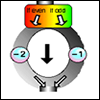The Number Crunching Machine

Age 7 to 11 Challenge Level:

Put a number at the top of the machine and collect a number at the bottom. What do you get? Which numbers get back to themselves?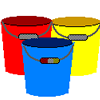Buckets of Thinking

Age 7 to 11 Challenge Level:

There are three buckets each of which holds a maximum of 5 litres. Use the clues to work out how much liquid there is in each bucket.X Is 5 Squares

Age 7 to 11 Challenge Level:

Can you arrange 5 different digits (from 0 - 9) in the cross in the way described?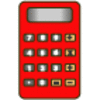Clever Keys

Age 7 to 11 Short Challenge Level:

On a calculator, make 15 by using only the 2 key and any of the four operations keys. How many ways can you find to do it?Arranging the Tables

Age 7 to 11 Challenge Level:

There are 44 people coming to a dinner party. There are 15 square tables that seat 4 people. Find a way to seat the 44 people using all 15 tables, with no empty places.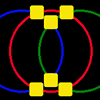Magic Circles

Age 7 to 11 Challenge Level:

Put the numbers 1, 2, 3, 4, 5, 6 into the squares so that the numbers on each circle add up to the same amount. Can you find the rule for giving another set of six numbers?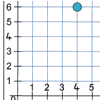Two Number Lines

Age 7 to 11 Challenge Level:

Max and Mandy put their number lines together to make a graph. How far had each of them moved along and up from 0 to get the counter to the place marked?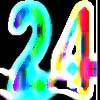Special 24

Age 7 to 11 Challenge Level:

Find another number that is one short of a square number and when you double it and add 1, the result is also a square number.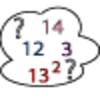Seven Square Numbers

Age 7 to 11 Challenge Level:

Add the sum of the squares of four numbers between 10 and 20 to the sum of the squares of three numbers less than 6 to make the square of another, larger, number.Diagonal in a Spiral

Age 7 to 11 Challenge Level:

Investigate the totals you get when adding numbers on the diagonal of this pattern in threes.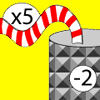Function Machines

Age 7 to 11 Challenge Level:

If the numbers 5, 7 and 4 go into this function machine, what numbers will come out?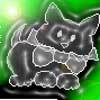Christmas Shopping

Age 7 to 11 Challenge Level:

Vera is shopping at a market with these coins in her purse. Which things could she give exactly the right amount for?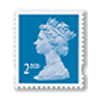Penta Post

Age 7 to 11 Challenge Level:

Here are the prices for 1st and 2nd class mail within the UK. You have an unlimited number of each of these stamps. Which stamps would you need to post a parcel weighing 825g?The Pied Piper of Hamelin

Age 7 to 11 Challenge Level:

This problem is based on the story of the Pied Piper of Hamelin. Investigate the different numbers of people and rats there could have been if you know how many legs there are altogether!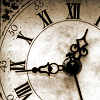Clock Face

Age 7 to 11 Challenge Level:

Where can you draw a line on a clock face so that the numbers on both sides have the same total?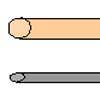A Rod and a Pole

Age 7 to 11 Challenge Level:

A lady has a steel rod and a wooden pole and she knows the length of each. How can she measure out an 8 unit piece of pole?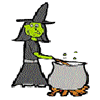Hubble, Bubble

Age 7 to 11 Challenge Level:

Winifred Wytsh bought a box each of jelly babies, milk jelly bears, yellow jelly bees and jelly belly beans. In how many different ways could she make a jolly jelly feast with 32 legs?